# KSEEB Solutions for Class 6 Maths Chapter 1 Knowing Our Numbers Ex 1.2

Students can Download Chapter 1 Knowing Our Numbers Ex 1.2 Questions and Answers, Notes Pdf, KSEEB Solutions for Class 6 Maths helps you to revise the complete Karnataka State Board Syllabus and score more marks in your examinations.

## Karnataka State Syllabus Class 6 Maths Chapter 1 Knowing Our Numbers Ex 1.2

Question 1.
A book exhibition was held for four days in a school. The number of tickets sold at the counter on the first, second, third, and final day was respectively 1094,1812, 2050, and 2751. Find the total number of tickets sold on all four days.
Solution:
Tickets sold on 1st day = 1094
Tickets sold on 2nd day = 1812
Tickets sold on 3rd day = 2050
Tickets sold on 4th day = 2751
Total tickets sold = 1094 + 1812 + 2050 + 2751 = 7,707

Question 2.
Shekhar is a famous cricket player. He has so far scored 6980 runs in test matches, He wishes to complete 10,000 runs. How many more runs does he need?
Solution:
Runs scored so far = 6980
Runs shekhar wants to score = 10,000
More runs required = 10,000 – 6,980 = 3,020
Shekhar required 3,020 more runs.Question 3.
In an election, the successful candidate registered 5,77,500 votes and his nearest rival secured 3,48,700 votes. By what margin did the successful candidate the election?
Solution:
Votes secured by successful candidate = 5,77,500
Votes secured by rival = 3,48,700
Margin = 5,77,500 – 3,48,700
Margin = 2,28,800.

Question 4.
Kirti bookstore sold books worth Rs2,85,891 in the first week of june and books worth Rs 4,00,768 in the second week of the month. How much was the sale for the two weeks together? In which week was the sale greater and by how much?
Solution:
Value of Books sold in 1st week = Rs 2,85,891
Value of books sold in 2nd week = Rs 4,00,768
Total sale = sale in 1st week + sale in 2nd week
= 2,85,891 + 4,00,768 = 6,86,659
The sale for the two weeks together was 6,86,659
Since 4,00,768 > 2,85,891, sale in 2nd week was greater than 1st week.
400768 – 285891 = 114877
The sale in 2nd week was larger than the sale in 1st week Rs 1,14,877

Question 5.
Find the difference between the greatest and the least number that can be written using the digits 6,2,7,4,3 each only once.
Solution:
Greatest number = 76432
Smallest number = 23467
Difference = 76432 – 23467
= 52965
There fore, the difference between the greatest and the least number that can the written using the digits 6, 2, 7, 4, 3 each only once is 52,965.Question 6.
A machine, on an average, manufactures 2,825 screws a day. How many screws did it produce in the month of January 2006?
Solution:
Screws produced in one day = 2,825
Days in January = 31
Screws produced in 31 days = 2825 × 31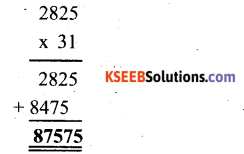Therefore, Screws produced during, Jan 06 = 87,575Question 7.
A merchant, had Rs 78,592 with her. She placed an order for purchasing 40 radio sets at Rs 1200 each. How much money will remain with her after the purchase?
Solution:
A merchant had Rs. 78,592 with her
Cost of one radio set = Rs 1200
Cost of 40 radio sets = 1200 × 40 = Rs 48000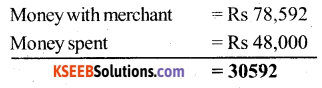Therefore Rs 30,592 will remain with her after the purchase.

Question 8.
A Student multiplied 7236 by 65 instead of multiplying by 56. By how much was his answer greater than the correct answer? (Hint: Do you need to do both the multi-plication?)
Solution:
Difference between 65 and 56 =9
difference in the answer = 7236 × 9
= 65124
OR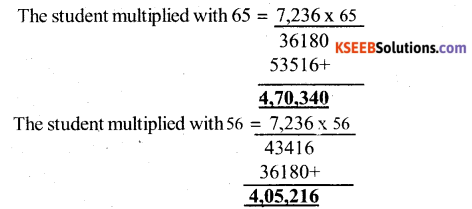The difference between (1) & (2) is 65124

Question 9.
To stitch a shirt, 2 ml5 cm cloth is needed, out of 40 m cloth, how many shirts can be stitched and how much cloth will remain?
(Hint: convert data in cm.)
Solution:
2m 15cm = 215 cm( 1 m = 100 cm)
40m = 40 × 100
= 4000 cm
cloth required for one shirt = 215 cm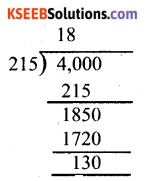Number of shirt that can be stitched out of 4000 cm = 4000 ÷ 215
therefore, is shirts can be made 130 cm, i,e, 1 m 30 cm, cloth will remain.Question 10.
Medicine is packed in boxes, each weighing 4 kg 500g. How many such boxes can be loaded in a van which cannot carry beyond 800 kg?
Solution:
1 kg = 1000 g
4 kg 500 g = 4500 g
800 kg = 80 × 1000 = 800000 g
Number of boxes that can loaded in the van = 800000 ÷ 4500 = 800 ÷ 4.5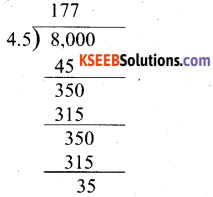Hence, 117 boxes at maximum can be loaded in the van

Question 11.
The distance between the school and the house of a student’s house is 1 km 875m. every day she walks both ways. Find the total distance covered by her in six days.
Solution:
Distance between school and house = 1 km 875 m
Now, 1 km = 1000 m
1 km 875 m = 1875 m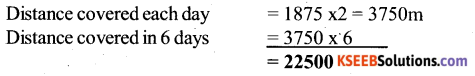Therefore, she covered total distance covered in 6 days = 22,500 m
= 22.5 km or 22 km 500m.Question 12.
A vessel has 4 liters and 500 ml of curd. In how many glasses, each of 25 ml capacity, can it be filled?
Solution:
Capacity of vessel = 4 l 500 ml
= 4500 ml (1 l = 100 ml)
capacity of a glasses that can be filled = 4500 ÷ 25
180 glasses can be filled